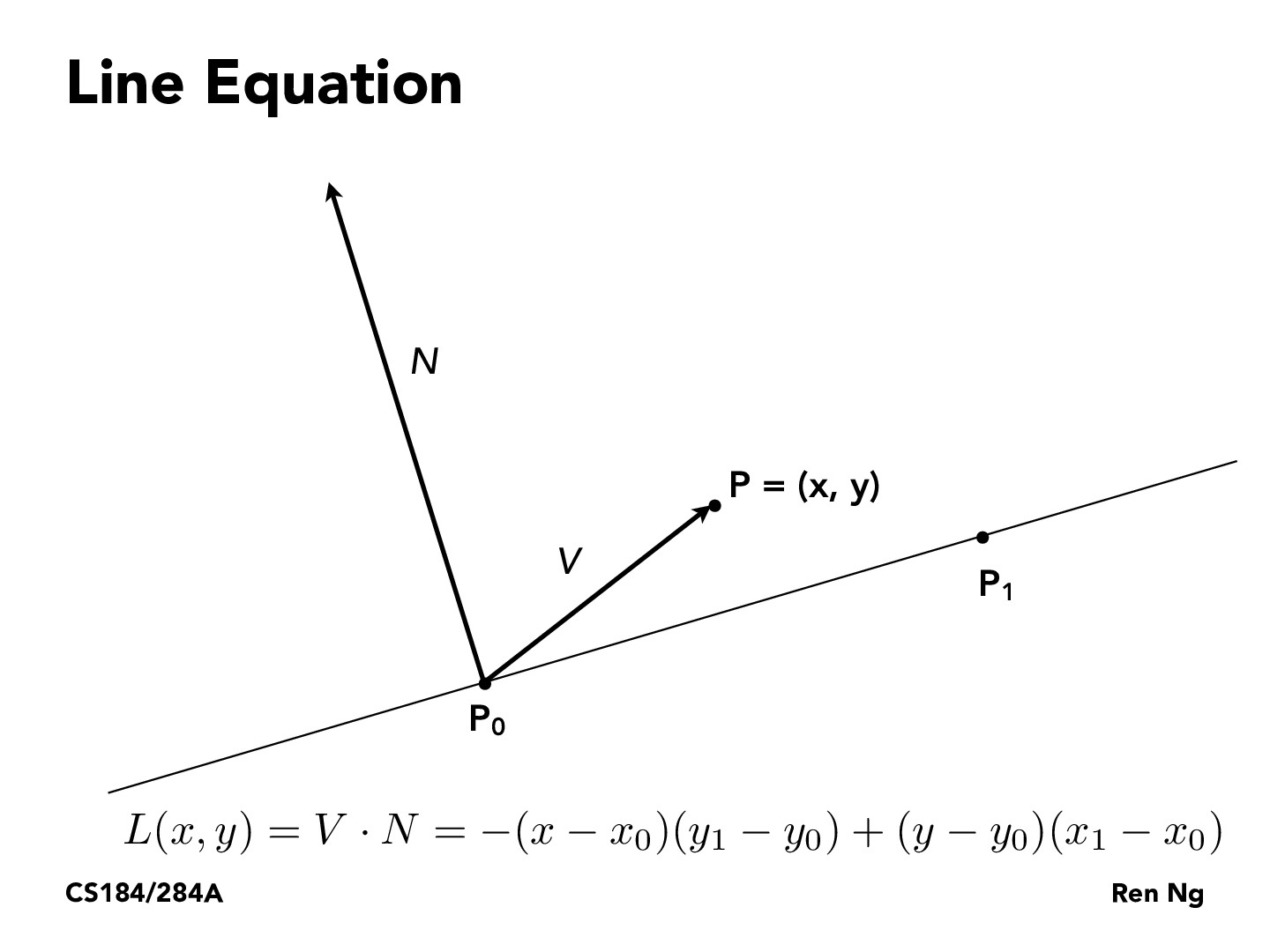Lecture 2: Sampling Triangles (42)JiaweiChenKodomo

Or we can use cross product of vector $\bar{P_0P_1}$ and $\bar{P_0P}$:

\begin{align*} \bar{P_0 P_1} \times \bar{P_0P} &= (x_1 - x_0, y_1 - y_0, 0) \times (x - x_0, y - y_0, 0) \ &= (0, 0, (x_1 - x_0)(y - y_0) - (x - x_0)(y_1 - y_0)). \end{align*}

$L(x,y)$ is the third component of the result.

You must be enrolled in the course to comment